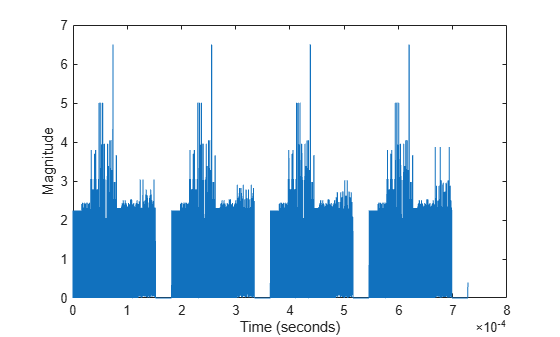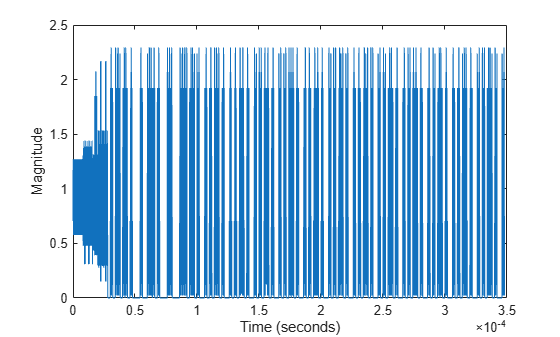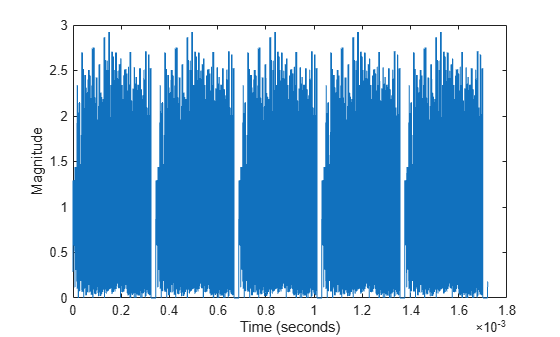# Waveform Generation

This example shows how to generate standards-compliant WLAN waveforms. The IEEE® 802.11™ standards define a physical layer protocol data unit (PPDU) as the transmission unit at the physical layer. For a detailed description of the PPDU field structures for each transmission format, see WLAN PPDU Structure.

### Generate WLAN Waveforms

#### Generate HE SU Waveform

Specify information bits to transmit.

`bits = [1 0 0 1 1];`

Parameterize the transmission by creating a high-efficiency single-user (HE SU) configuration object with default property values.

`cfgHESU = wlanHESUConfig;`

Specify an oversampling factor for fast-Fourier-transform-based oversampling.

`osf = 2;`

Generate an HE SU waveform, as defined in , for the specified bits and transmission parameters. The waveform comprises four packets with 15 microseconds of idle time between them.

```waveformHESU = wlanWaveformGenerator(bits,cfgHESU, ... NumPackets=4,IdleTime=15e-6, ... OversamplingFactor=osf);```

Plot the HE SU waveform, scaling the x-axis relative to the channel bandwidth.

```fsHESU = wlanSampleRate(cfgHESU.ChannelBandwidth); time = (0:length(waveformHESU)-1)/fsHESU; plot(time,abs(waveformHESU)) xlabel ("Time (seconds)") ylabel("Magnitude")```#### Generate HE MU Waveform

Parameterize the transmission by creating an HE multi-user (HE MU) configuration object.

`cfgHEMU = wlanHEMUConfig(192);`

Generate an HE MU waveform, as defined in , comprising three packets with 30 microseconds of idle time between packets for the specified bits and transmission parameters.

```waveformHEMU = wlanWaveformGenerator(bits,cfgHEMU, ... NumPackets=3,IdleTime=30e-6);```

Plot the HE MU waveform, scaling the x-axis relative to the channel bandwidth.

```fsHEMU = wlanSampleRate(cfgHEMU.ChannelBandwidth); time = (0:length(waveformHEMU)-1)/fsHEMU; plot(time,abs(waveformHEMU)) xlabel ("Time (seconds)") ylabel("Magnitude")```#### Generate WUR Waveform

Parameterize the transmission by creating a wake-up radio (WUR) configuration object.

`cfgWUR = wlanWURConfig(ChannelBandwidth="CBW40",NumUsers=2);`

Generate a single-packet WUR waveform, as defined in , for the specified bits and transmission parameters.

`waveformWUR = wlanWaveformGenerator(bits,cfgWUR);`

Plot the WUR waveform, scaling the x-axis relative to the channel bandwidth.

```fsWUR = wlanSampleRate(cfgWUR.ChannelBandwidth); time = (0:length(waveformWUR)-1)/fsWUR; plot(time,abs(waveformWUR)) xlabel ("Time (seconds)") ylabel("Magnitude")```#### Generate VHT Waveform

Parameterize the transmission by creating a VHT configuration object with default property values.

`cfgVHT = wlanVHTConfig;`

Generate a VHT waveform, as defined in , for the specified bits and transmission parameters. The waveform comprises five packets with 20 microseconds of idle time between them.

```waveformVHT = wlanWaveformGenerator(bits,cfgVHT, ... NumPackets=5,IdleTime=20e-6);```

Plot the VHT waveform, scaling the x-axis relative to the channel bandwidth.

```fsVHT = wlanSampleRate(cfgVHT.ChannelBandwidth); time = (0:length(waveformVHT)-1)/fsVHT; plot(time,abs(waveformVHT)) xlabel ('Time (seconds)') ylabel('Magnitude')```### Generate Waveforms of Individual PPDU Fields

You can also create a VHT, HT, or non-HT PPDU waveform by generating and concatenating waveforms for individual PPDU fields. For an example of this procedure, see Build VHT PPDU.

This list shows which functions you can use to build a PPDU for each PHY format.